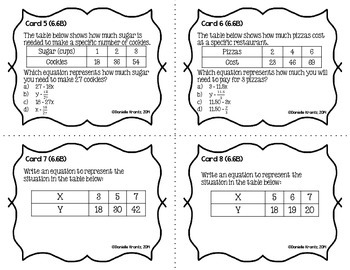STAAR Prep Math Task Cards Expressions, Equations, and Relationships - Grade 6Subject
Resource Type
Product Rating
File Type

PDF (Acrobat) Document File

2 MB|20 pages
Share
Product Description
These have been UPDATED to reflect the new TEKS!

This is a set of 64 task cards to review the Expressions, Equations, and Relationships strand with your students before the STAAR test. The cards cover the following TEKS (4 questions per TEK):

(6.6)  The student applies mathematical process standards to use multiple representations to describe algebraic relationships. The student is expected to:
-(6.6A)  identify independent and dependent quantities from tables and graphs;
-(6.6B)  write an equation that represents the relationship between independent and dependent quantities from a table; and
-(6.6C)  represent a given situation using verbal descriptions, tables, graphs, and equations in the form y = kx or y = x + b.
(6.7)  The student applies mathematical process standards to develop concepts of expressions and equations. The student is expected to:
-(6.7A)  generate equivalent numerical expressions using order of operations, including whole number exponents and prime factorization;
-(6.7B)  distinguish between expressions and equations verbally, -numerically, and algebraically;
-(6.7C)  determine if two expressions are equivalent using concrete models, pictorial models, and algebraic representations; and
-(6.7D)  generate equivalent expressions using the properties of operations: inverse, identity, commutative, associative, and distributive properties.
(6.8)  The student applies mathematical process standards to use geometry to represent relationships and solve problems. The student is expected to:
-(6.8A)  extend previous knowledge of triangles and their properties to include the sum of angles of a triangle, the relationship between the lengths of sides and measures of angles in a triangle, and determining when three lengths form a triangle;
-(6.8B)  model area formulas for parallelograms, trapezoids, and triangles by decomposing and rearranging parts of these shapes;
-(6.8C)  write equations that represent problems related to the area of rectangles, parallelograms, trapezoids, and triangles and volume of right rectangular prisms where dimensions are positive rational numbers; and
-(6.8D)  determine solutions for problems involving the area of rectangles, parallelograms, trapezoids, and triangles and volume of right rectangular prisms where dimensions are positive rational numbers.
(6.9)  The student applies mathematical process standards to use equations and inequalities to represent situations. The student is expected to:
-(6.9A)  write one-variable, one-step equations and inequalities to represent constraints or conditions within problems;
-(6.9B)  represent solutions for one-variable, one-step equations and inequalities on number lines; and
-(6.9C)  write corresponding real-world problems given one-variable, one-step equations or inequalities.
(6.10)  The student applies mathematical process standards to use equations and inequalities to solve problems. The student is expected to:
-(6.10A)  model and solve one-variable, one-step equations and inequalities that represent problems, including geometric concepts; --(6.10B)  determine if the given value(s) make(s) one-variable, one-step equations or inequalities true.

Most are also dual-coded to include the process standards as well (6.1A-G)

This is now bundled with the other reporting categories for 6th grade and can be found here: STAAR Prep Task Card Bundle for 6th Grade

This set of task cards has been created without a background image to save ink! Simply print on colored cardstock and laminate for continued use each year.

You can find more STAAR PRACTICE here: More STAAR Prep

Please note: This file WILL be updated to align with the new TEKS revision in 2014. By purchasing now, you will get the revised file FOR FREE!

This is also included in my Complete Bundle of Task Cards

*****************************************************************************
Important Information for Customers:

How to get TPT credit to use on future purchases:
• Please go to your My Purchases page (you may need to login). Under each purchase you'll see a Provide Feedback button. Simply click it and you will be taken to a page where you can give a quick rating and leave a short comment for the product. Each time you give feedback, TPT gives you feedback credits that you may redeem on future purchases. I value your feedback and use it to create future products for you to use with your classes!

Be the first to know about my new discounts, freebies and product launches:
• Look for the green star next to my store logo and click it to become a follower. You will now receive email updates and be the first to know about new products and sales.

You may also be interested in following me on:
My Blog
Instagram
Pinterest

If you have any questions, please feel free to email me at livelovemathtpt@gmail.com
Total Pages
20 pages